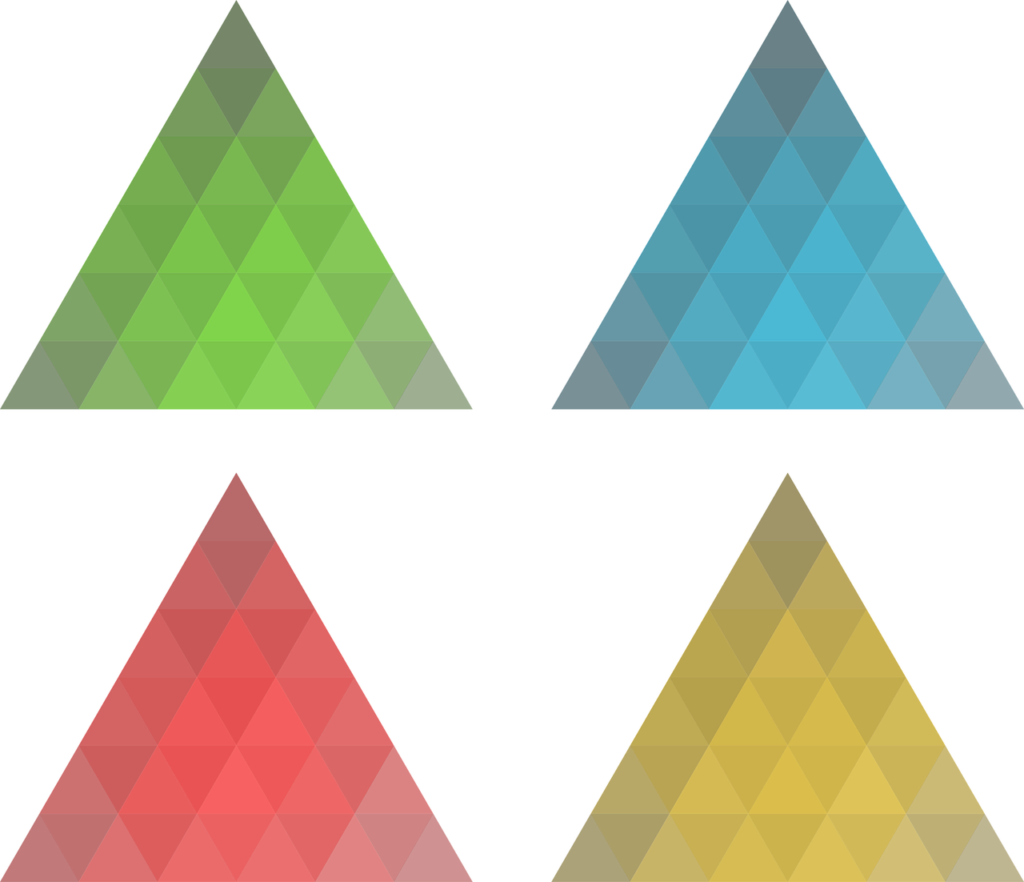## The Similarity of Triangles Class 10th

Introduction The triangle is the shape, we are well known about it and its properties. We have studied different triangles and terms related to them like a scalene triangle, equilateral triangle, isosceles triangle, area of the triangle, the perimeter of a triangle, the median of a triangle, altitude of a triangle, etc. In the previous …